• +91 9971497814
• info@interviewmaterial.com

# RD Chapter 12- Heron-s Formula Ex-12.1 Interview Questions Answers

### Related Subjects

Question 1 : In the figure, the sides BA and CA have been produced such that BA = AD and CA = AE. Prove the segment DE || BC.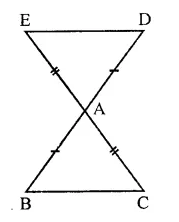Given : Sides BA and CA of ∆ABC are produced such that BA = AD are CA = AE. ED is joined.
To prove : DE || BC
Proof: In ∆ABC and ∆DAE AB=AD (Given)
AC = AE (Given)
∠BAC = ∠DAE (Vertically opposite angles)
∴ ∆ABC ≅ ∆DAE (SAS axiom)
But there are alternate angles
∴ DE || BC

Question 2 : In a ∆PQR, if PQ = QR and L, M and N are the mid-points of the sides PQ, QR and RP respectively. Prove that LN = MN.

Given : In ∆PQR, PQ = QR
L, M and N are the mid points of the sides PQ, QR and PR respectively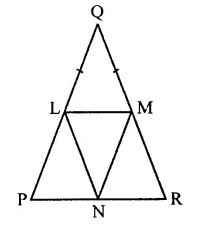To prove : LM = MN
Proof : In ∆LPN and ∆MRH
PN = RN (∵ M is mid point of PR)
LP = MR (Half of equal sides)
∠P = ∠R (Angles opposite to equal sides)
∴ ALPN ≅ AMRH (SAS axiom)
∴ LN = MN (c.p.c.t.)

Question 3 : Prove that the medians of an equilateral triangle are equal.

Given : In ∆ABC, AD, BE and CF are the medians of triangle and AB = BC = CA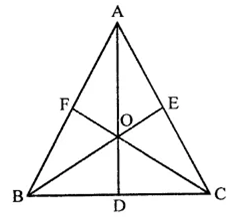To prove : AD = BE = CF
Proof : In ∆BCE and ∆BCF,
BC = BC (Common side)
CE = BF (Half of equal sides)
∠C = ∠B (Angles opposite to equal sides)
∴ ABCE ≅ ABCF (SAS axiom)
∴ BE = CF (c.p.c.t.) …(i)
Similarly, we can prove that
From (i) and (ii)
⇒ AD = BE = CF

Question 4 : In a ∆ABC, if ∠A = 120° and AB = AC. Find ∠B and ∠C.

In ∆ABC, ∠A = 120° and AB = AC
∴ ∠B = ∠C (Angles opposite to equal sides)
But ∠A + ∠B + ∠C = 180° (Sum of angles of a triangle)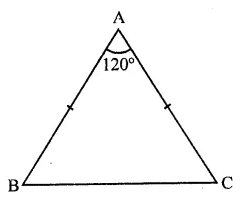⇒ 120° + ∠B + ∠B = 180°
⇒ 2∠B = 180° – 120° = 60°
∴ ∠B =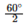= 30°
and ∠C = ∠B = 30°
Hence ∠B = 30° and ∠C = 30°

Question 5 : In a ∆ABC, if AB = AC and ∠B = 70°, find ∠A.

In ∆ABC, ∠B = 70°
AB =AC
∴ ∠B = ∠C (Angles opposite to equal sides)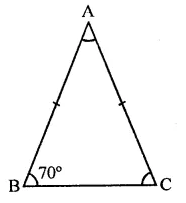But ∠B = 70°
∴ ∠C = 70°
But ∠A + ∠B + ∠C = 180° (Sum of angles of a triangle)
⇒ ∠A + 70° + 70° = 180°
⇒ ∠A + 140°= 180°
∴∠A = 180°- 140° = 40°

Question 6 : The vertical angle of an isosceles triangle is 100°. Find its base angles.

In ∆ABC, AB = AC and ∠A = 100°
But AB = AC (In isosceles triangle)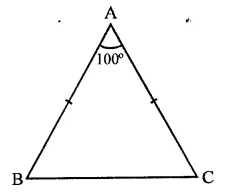∴ ∠C = ∠B (Angles opposite to equal sides)
∠A + ∠B + ∠C = 180° (Sum of angles of a triangle)
⇒ 100° + ∠B + ∠B = 180° (∵ ∠C = ∠B)
⇒ 2∠B = 180° – 100° = 80°
∴ ∠C = ∠B = 40°
Hence ∠B = 40°, ∠C = 40°

Question 7 : In the figure, AB = AC and ∠ACD = 105°, find ∠BAC.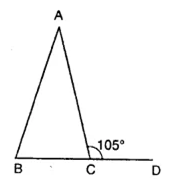In ∆ABC, AB = AC
∴ ∠B = ∠C (Angles opposite to equal sides)
But ∠ACB + ∠ACD = 180° (Linear pair)
⇒ ∠ACB + 105°= 180°
⇒ ∠ACB = 180°-105° = 75°
∴ ∠ABC = ∠ACB = 75°
But ∠A + ∠B + ∠C = 180° (Sum of angles of a triangle)
⇒ ∠A + 75° + 75° = 180°
⇒ ∠A + 150°= 180°
⇒ ∠A= 180°- 150° = 30°
∴ ∠BAC = 30°

Question 8 : Find the measure of each exterior angle of an equilateral triangle.

In an equilateral triangle, each interior angle is 60°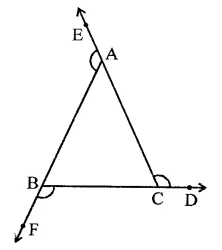But interior angle + exterior angle at each vertex = 180°
∴ Each exterior angle = 180° – 60° = 120°

Question 9 : If the base of an isosceles triangle is produced on both sides, prove that the exterior angles so formed are equal to each other.

Given : In an isosceles ∆ABC, AB = AC
and base BC is produced both ways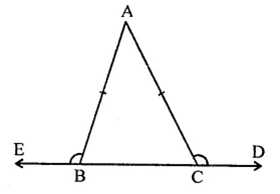To prove : ∠ACD = ∠ABE
Proof: In ∆ABC,
∵ AB = AC
∴∠C = ∠B (Angles opposite to equal sides)
⇒ ∠ACB = ∠ABC
But ∠ACD + ∠ACB = 180° (Linear pair)
and ∠ABE + ∠ABC = 180°
∴ ∠ACD + ∠ACB = ∠ABE + ∠ABC
But ∠ACB = ∠ABC (Proved)
∴ ∠ACD = ∠ABE
Hence proved.

Question 10 : In the figure, AB = AC and DB = DC, find the ratio ∠ABD : ∠ACD.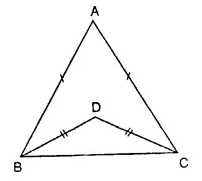In the given figure,
In ∆ABC,
AB = AC and DB = DC
In ∆ABC,
∵ AB = AC
∴ ∠ACD = ∠ABE …(i) (Angles opposite to equal sides)
Similarly, in ∆DBC,
DB = DC
∴ ∠DCB = ∠DBC .. (ii)
Subtracting (ii) from (i)
∠ACB – ∠DCB = ∠ABC – ∠DBC
⇒ ∠ACD = ∠ABD
∴ Ratio ∠ABD : ∠ACD = 1 : 1

Todays Deals### RD Chapter 12- Heron-s Formula Ex-12.1 Contributorskrishan

Name:
Email:

# Latest News# 9000 interview questions in different categories